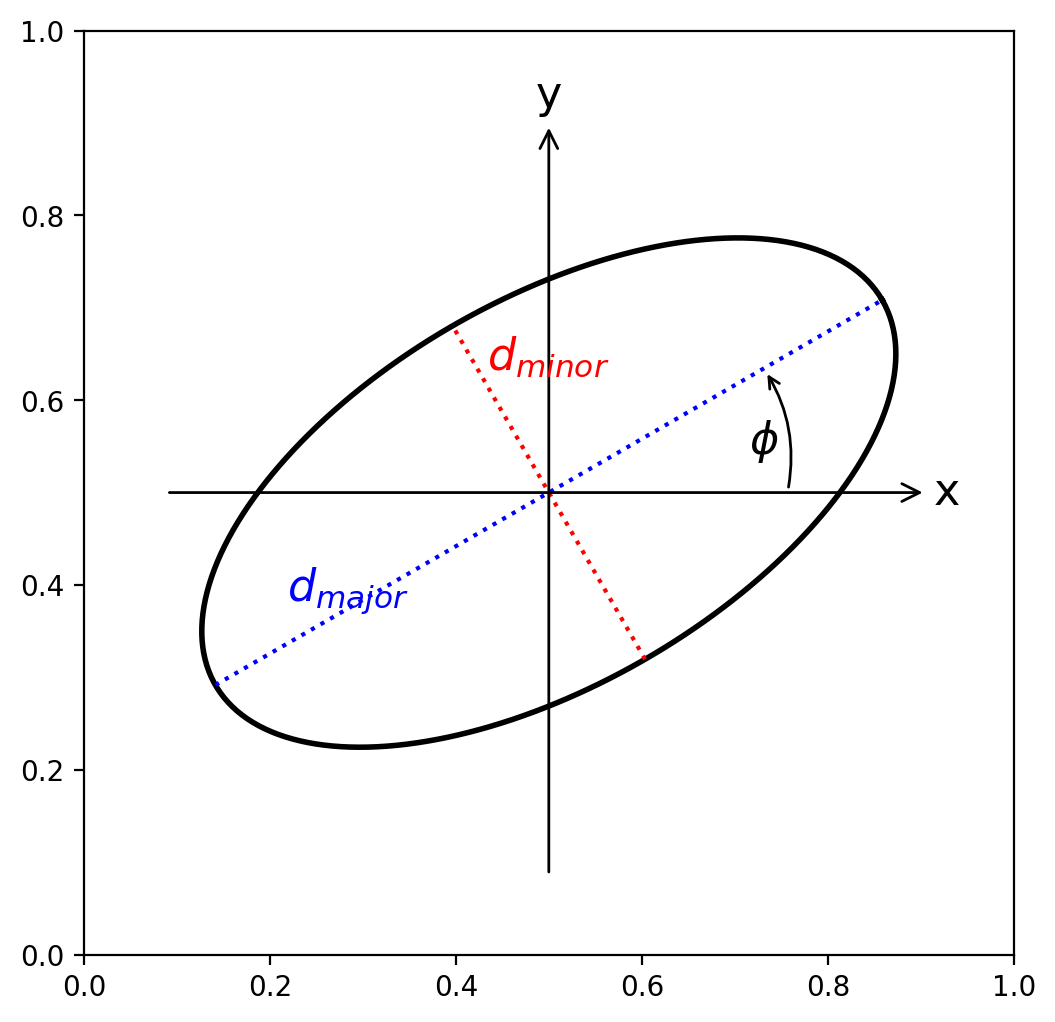# ISO 11146 Beam Size Definitions

Scott Prahl

Sept 2023

A laser beam will usually consist of a superposition of several modes. A single mode beam radius is easily described by $$1/e^2$$ points. This, of course, fails for any beam shape other than Gaussian. ISO 11146 is intended to provide a simple, consistent way of describing the width of a beam.

This notebook summarizes the mathematical definitions.

:

import numpy as np
import matplotlib.pyplot as plt
import laserbeamsize as lbs

:

lbs.plot_beam_diagram()The total power $$P$$ is obtained by integrating the irradiance $$E(x,y)$$ over the entire beam

$P = \int_{-\infty}^\infty \int_{-\infty}^\infty E(x,y)\,dxdy$

## Center of beam

The center of the beam can be found by

$x_c = {1\over P} \int_{-\infty}^\infty \int_{-\infty}^\infty x \cdot E(x,y)\,dxdy$

and

$y_c = {1\over P} \int_{-\infty}^\infty \int_{-\infty}^\infty y \cdot E(x,y)\,dxdy$

## Variance

A useful parameter characterizing a general two-dimensional distribution $$E(x,y)$$ is the variance in the $$x$$ and $$y$$ directions

$\sigma_x^2 = {1\over P} \int_{-\infty}^\infty \int_{-\infty}^\infty (x-x_c)^2 E(x,y)\,dxdy$

and

$\sigma_y^2 = {1\over P} \int_{-\infty}^\infty \int_{-\infty}^\infty (y-y_c)^2 E(x,y)\,dxdy$

and

$\sigma_{xy}^2 = {1\over P} \int_{-\infty}^\infty \int_{-\infty}^\infty (x-x_c)(y-y_c) E(x,y)\,dxdy$

In general, $$\sigma_x \ne \sigma_y$$. For example, in semiconductor lasers the height and width of the emitting aperture differ. Such beams are called astigmatic.

Now, the quantities $$\sigma_x^2$$ and $$\sigma_y^2$$ will always be positive, but $$\sigma_{xy}^2$$ can be negative, zero, or positive.

## Beam Radius and $$D4\sigma$$ or D4sigma

For a Gaussian distribution centered at (0,0) with $$1/e^2$$ radius $$w$$ we find

$\sigma_x^2 = \frac{\int_{-\infty}^\infty \int_{-\infty}^\infty x^2 e^{-2(x^2+y^2)/w^2}\,dx\,dy}{ \int_{-\infty}^\infty \int_{-\infty}^\infty e^{-2(x^2+y^2)/w^2}\,dx\,dy} =\frac{w^2}{4}$

$w_x = 2\sigma_x \qquad w_y = 2\sigma_y$

## $$D4\sigma$$ or D4sigma

The $$D4\sigma$$ beam diameter is a simple rearrangement of the above equation in which it is noted that twice the radius or the beam diameter is equal to $$4\sigma$$

$4\sigma_x = 2w_x$

### Relationship to FWHM

Sometimes it is the full width at half maximum (FWHM) value that is desired or known. In general, there is no direct relationship between the $$1/e^2$$ radius $$w$$ and the FWHM. However, in the special case of a Gaussian beam, there is.

$w={ \mathrm {FWHM}\over \sqrt {2\ln 2}}$

### Major and minor axes of an elliptical beam

The $$x$$-axis diameter $$d_x=2w_x$$ is given by

$d_x = \sqrt{8(\sigma_x^2 + \sigma_y^2) + 8\operatorname{sign}(\sigma_x^2 -\sigma_y^2) \sqrt{(\sigma_x^2 -\sigma_y^2)^2+4\sigma_{xy}^4}}$

and similarly $$d_y=2w_y$$ is

$d_y = \sqrt{8(\sigma_x^2 + \sigma_y^2) - 8\operatorname{sign}(\sigma_x^2 -\sigma_y^2) \sqrt{(\sigma_x^2 -\sigma_y^2)^2+4\sigma_{xy}^4}}$

except if $$\sigma_x^2=\sigma_y^2$$ in which case

$d_x = \sqrt{8\sigma_x^2 + 8\sigma_y^2 + 16|\sigma_{xy}^2|}$

and

$d_y = \sqrt{8\sigma_x^2 + 8\sigma_y^2 - 16|\sigma_{xy}^2|}$

### The tilt angle of the ellipse $$\phi$$

This is measured as a positive angle counter-clockwise from the $$x$$-axis (see figure above).

$\phi =\frac{1}{2}\arctan \frac{2\sigma_{xy}}{\sigma_x^2 -\sigma_y^2}$

where we use np.arctan2(numerator,denominator) to avoid division by zero when $$\sigma_x^2=\sigma_y^2$$

### Ellipticity and Eccentricity

The ellipticity $$e$$ is

$\varepsilon = \frac{\operatorname{min}(dx,dy)}{\operatorname{max}(dx,dy)}$

and the eccentricity is

$e = \sqrt{1-\varepsilon^2}$